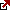# Formula for Ending Balance with Compound Interest

Compound interest plays a pivotal role in finance and investments, often dubbed the “eighth wonder of the world” by financiers and mathematicians alike. It signifies the interest calculated on the initial principal amount, including all the accumulated interest from previous periods on a deposit or loan.

## What is Compound Interest?

Compound interest is the formula calculating the interest earned on trading or investing accounts where the amount earned is reinvested. The “compound” term is used because the investor will reinvest the earned amount.

Compound interest is one of the most excellent tools you can utilize to build wealth. First of all, what exactly is compound interest? Usually, you would make a percentage return on it, known as simple interest. Compound interest is where you keep earning money on your principal balance and the money you made as simple interest. This means that your money will keep multiplying, and it will start to grow exponentially after a while.

## Formula for Ending Balance with Compound Interest

C= P[(1+r)^n – 1]

The definitions of these variables are listed below

C = Compound interest

P = Principle (the original balance)

r = Interest rate per period

n = The number of periods

For you to fully understand how to utilize this formula, I will demonstrate it using an example.

### Critical Components of the Compound Interest Formula

1. C – Compound Interest: This is the interest earned or paid on the Principal over a specified period, minus the Principal itself. It represents the extra money that grows in an investment due to the ‘interest on interest’ effect.
2. P – Principal: This is the initial amount of money you invest or deposit, or in the context of loans, the amount borrowed.
3. r – Interest Rate per Period: This is the rate at which interest accrues for each period. Note that it’s crucial to match the rate with the period. For example, if you’re calculating yearly compound interest but have a monthly rate, you’d need to adjust it accordingly.
4. n – Number of Periods: This represents the number of times interest is applied to the Principal. If you have a yearly compound interest but have invested the money for five years, n would be 5.

### Illustrative Example

Imagine investing $1,000 in a bank account (Principal, P) offering a 5% annual compound interest (rate, r). You plan to leave the money in the account for 3 years (number of periods, n). Plugging these values into the formula: $=1000\left[\left(1+0.05{\right)}^{3}?1\right]$ $=1000\left[1.157625?1\right]$ $=1000\left[0.157625\right]$ C =$157.63

So, after three years, you will earn a compound interest of $157.63. Your total balance at the end of the period would be the Principal + Compound Interest, i.e.,$1,000 + $157.63 =$1,157.63.

### Significance of Compound Interest

The magic of compound interest lies in the exponential growth of the investment. The end amount can be substantial even with small principal amounts, given enough time and a decent rate. This is the Principle upon which long-term investments, retirement funds, and savings accounts operate. It’s the reason financial advisors consistently emphasize starting investments early: the longer your money has to compound, the more you stand to gain.

In conclusion, the compound interest formula provides a mathematical representation of the growth potential of an investment or the potential costs of a loan when interest is compounded. Understanding and harnessing the power of this formula can lead to smarter financial decisions and a more precise grasp of the time value of money.

## Calculating Compound Interest

Suppose you take $1000 and put it into an investment. Your rate of return is 10% and is compounded annually. I will use this formula to determine your balance after 20 years. So, how to solve this: Use the simple interest to find the ending balance of$1000 at 4% for 20 years!

Step 1

Plug the variables into the formula

C = Unknown

P = $1000 r = 10% The interest rate will be converted into a decimal, so 0.1 will be plugged into the formula n = 20 Here is the formula with all of the variables substituted C =$1000[(1 + 0.1)^20 – 1]

The last step is to plug this into your calculator! After plugging this into my calculator, I have determined that the final balance under these conditions will be roughly $5728. If you were to use the same conditions while earning simple interest, your final balance would be$2000 after 20 years. As you can see, there is a significant difference in the earning potential between compound and simple interest.

Latest posts by Fxigor (see all)

Fxigor

Igor has been a trader since 2007. Currently, Igor works for several prop trading companies. He is an expert in financial niche, long-term trading, and weekly technical levels. The primary field of Igor's research is the application of machine learning in algorithmic trading. Education: Computer Engineering and Ph.D. in machine learning. Igor regularly publishes trading-related videos on the Fxigor Youtube channel. To contact Igor write on: igor@forex.in.rs

Trade gold and silver. Visit the broker's page and start trading high liquidity spot metals - the most traded instruments in the world.Diversify your savings with a gold IRA.

Gold & silver portfolio-building assistance from product professionals.

If you want to trade stocks try: